Study Materials: ncert solutions

Our ncert solutions for Exercise 1.1 Class 8 maths 1. Rational Numbers - ncert solutions - Toppers Study is the best material for English Medium students cbse board and other state boards students.

Solutions ⇒ Class 8th ⇒ Mathematics ⇒ 1. Rational Numbers

# Exercise 1.1 Class 8 maths 1. Rational Numbers - ncert solutions - Toppers Study

Topper Study classes prepares ncert solutions on practical base problems and comes out with the best result that helps the students and teachers as well as tutors and so many ecademic coaching classes that they need in practical life. Our ncert solutions for Exercise 1.1 Class 8 maths 1. Rational Numbers - ncert solutions - Toppers Study is the best material for English Medium students cbse board and other state boards students.

## Exercise 1.1 Class 8 maths 1. Rational Numbers - ncert solutions - Toppers Study

CBSE board students who preparing for class 8 ncert solutions maths and Mathematics solved exercise chapter 1. Rational Numbers available and this helps in upcoming exams 2023-2024.

### You can Find Mathematics solution Class 8 Chapter 1. Rational Numbers

• All Chapter review quick revision notes for chapter 1. Rational Numbers Class 8
• NCERT Solutions And Textual questions Answers Class 8 Mathematics
• Extra NCERT Book questions Answers Class 8 Mathematics
• Importatnt key points with additional Assignment and questions bank solved.

NCERT Solutions do not only help you to cover your syllabus but also will give to textual support in exams 2023-2024 to complete exercise 1.1 maths class 8 chapter 1 in english medium. So revise and practice these all cbse study materials like class 8 maths chapter 1.1 in english ncert book. Also ensure to repractice all syllabus within time or before board exams for ncert class 8 maths ex 1.1 in english.

See all solutions for class 8 maths chapter 1 exercise 1 in english medium solved questions with answers.

### Exercise 1.1 class 8 Mathematics Chapter 1. Rational Numbers

• Exercise 1.1 Class 8 Maths 1. Rational Numbers - Ncert Solutions - Toppers Study
• Class 8 Ncert Solutions
• Solution Chapter 1. Rational Numbers Class 8
• Solutions Class 8
• Chapter 1. Rational Numbers Exercise 1.1 Class 8

## Exercise 1.1 Class 8 maths 1. Rational Numbers - ncert solutions - Toppers Study

Exercise 1.1

Q1. Using appropriate properties find;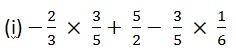Solution:Using BODMAS first we solve for multiply here;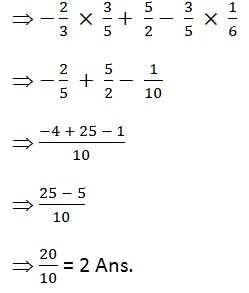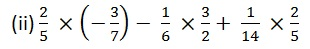Solution:Using BODMAS first we solve for multiply here;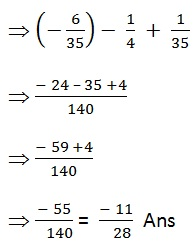Q2. Write the additive inverse of each of the following.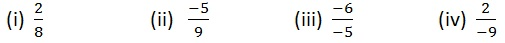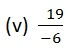Solution:Q3. Verify that – (- x) = x for;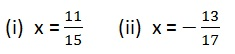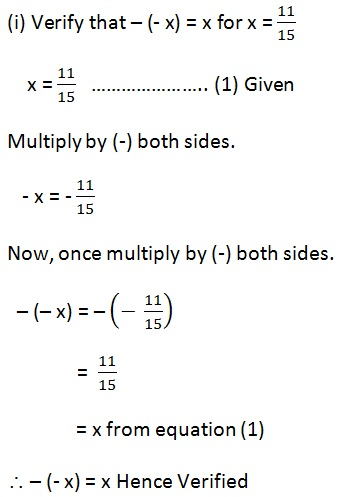##### Other Pages of this Chapter: 1. Rational Numbers

Important Study materials for classes 06, 07, 08,09,10, 11 and 12. Like CBSE Notes, Notes for Science, Notes for maths, Notes for Social Science, Notes for Accountancy, Notes for Economics, Notes for political Science, Noes for History, Notes For Bussiness Study, Physical Educations, Sample Papers, Test Papers, Mock Test Papers, Support Materials and Books.Mathematics Class - 11th

NCERT Maths book for CBSE Students.

books

## Study Materials List:

##### Solutions ⇒ Class 8th ⇒ Mathematics
1. Rational Numbers
2. Linear Equations in One Variable
4. Practical Geometry
5. Data Handling
6. Squares and Square Roots
7. Cubes and Cube Roots
8. Comparing Quantities
9. Algebraic Expressions and Identities
10. Visualising Solid Shapes
11. Mensuration
12. Exponents and Powers
13. Direct and Inverse Proportions
14. Factorisation
15. Introduction to Graphs
16. Playing with Numbers

New Books